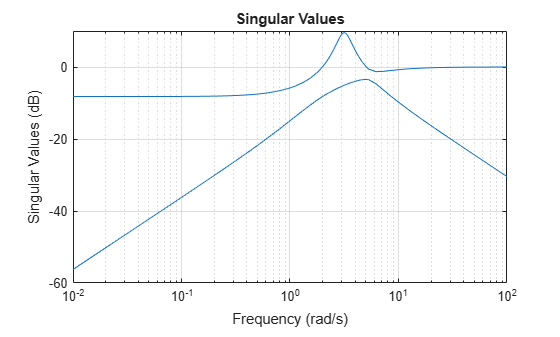Documentation

# hinfnorm

H norm of dynamic system

## Syntax

``ninf = hinfnorm(sys)``
``ninf = hinfnorm(sys,tol)``
``````[ninf,fpeak] = hinfnorm(___)``````

## Description

````ninf = hinfnorm(sys)` returns the H∞ norm in absolute units of the dynamic system model, `sys`. If `sys` is a stable SISO system, then the H∞ norm is the peak gain, the largest value of the frequency response magnitude.If `sys` is a stable MIMO system, then the H∞ norm is the largest singular value across frequencies.If `sys` is an unstable system, then the H∞ norm is defined as `Inf`.If `sys` is a model that has tunable or uncertain parameters, then `hinfnorm` evaluates the H∞ norm at the current or nominal value of `sys`.If is a model array, then `hinfnorm` returns an array of the same size as `sys`, where ```ninf(k) = hinfnorm(sys(:,:,k))``` .For stable systems, `hinfnorm(sys)` is the same as `getPeakGain(sys)`.```
````ninf = hinfnorm(sys,tol)` returns the H∞ norm of `sys` with relative accuracy `tol`. ```

example

``````[ninf,fpeak] = hinfnorm(___)``` also returns the frequency, `fpeak`, at which the peak gain or largest singular value occurs. You can use this syntax with any of the input arguments in previous syntaxes. If `sys` is unstable, then `fpeak` = `Inf`.```

## Examples

collapse all

Compute the ${H}_{\infty }$ norm of the following 2-input, 2-output dynamic system and the frequency at which the peak singular value occurs.

`$\mathit{G}\left(\mathit{s}\right)=\left[\begin{array}{cc}0& \frac{3\mathit{s}}{{\mathit{s}}^{2}+\mathit{s}+10}\\ \frac{\mathit{s}+1}{\mathit{s}+5}& \frac{2}{\mathit{s}+6}\end{array}\right].$`

```G = [0 tf([3 0],[1 1 10]);tf([1 1],[1 5]),tf(2,[1 6])]; [ninf,fpeak] = hinfnorm(G)```
```ninf = 3.0150 ```
```fpeak = 3.1623 ```

The ${H}_{\infty }$ norm of a MIMO system is its maximum singular value. Plot the singular values of `G` and compare the result from `hinfnorm`.

`sigma(G),grid`The values `ninf` and `fpeak` are consistent with the singular value plot, which displays the values in dB.

## Input Arguments

collapse all

Input dynamic system, specified as any dynamic system model or model array. `sys` can be SISO or MIMO.

Relative accuracy of the peak gain, specified as a positive real scalar value. `hinfnorm` calculates `ninf` such that the fractional difference between `ninf` and the true H norm of `sys` is no greater than `tol`.

## Output Arguments

collapse all

H norm of `sys`, returned as `Inf`, a scalar value, or an array.

• If `sys` is a single stable model, then `ninf` is a scalar value.

• If `sys` is a single unstable model, then `ninf` is `Inf`.

• If `sys` is a model array, then `ninf` is an array of the same size as `sys`, where ```ninf(k) = hinfnorm(sys(:,:,k))```.

Frequency at which the peak gain or largest singular value occurs, returned as `Inf`, a nonnegative real scalar value, or an array. The frequency is expressed in units of rad/`TimeUnit`, relative to the `TimeUnit` property of `sys`.

• If `sys` is a single stable model, then `fpeak` is a scalar.

• If `sys` is a single unstable model, then `fpeak` is `Inf`.

• If `sys` is a model array, then `fpeak` is an array of the same size as `sys`.In this case, `fpeak(k)` is the peak gain or largest singular value frequency of the kth model in the array.